# Time Series

library(fpp2)
library(zoo)
library(plotly)
require(gridExtra)

## Question 3.1

For the following series, find an appropriate Box-Cox transformation in order to stabilise the variance. usnetelec usgdp mcopper enplanements

#### usnetelec

data(usnetelec)
invisible(lambda <- round(BoxCox.lambda(usnetelec),3))
grid.arrange(autoplot(usnetelec) + ggtitle("usnetelec"),autoplot(BoxCox(usnetelec,lambda)) + ggtitle(paste("BoxCox Transform, lambda =", lambda)), ncol = 1)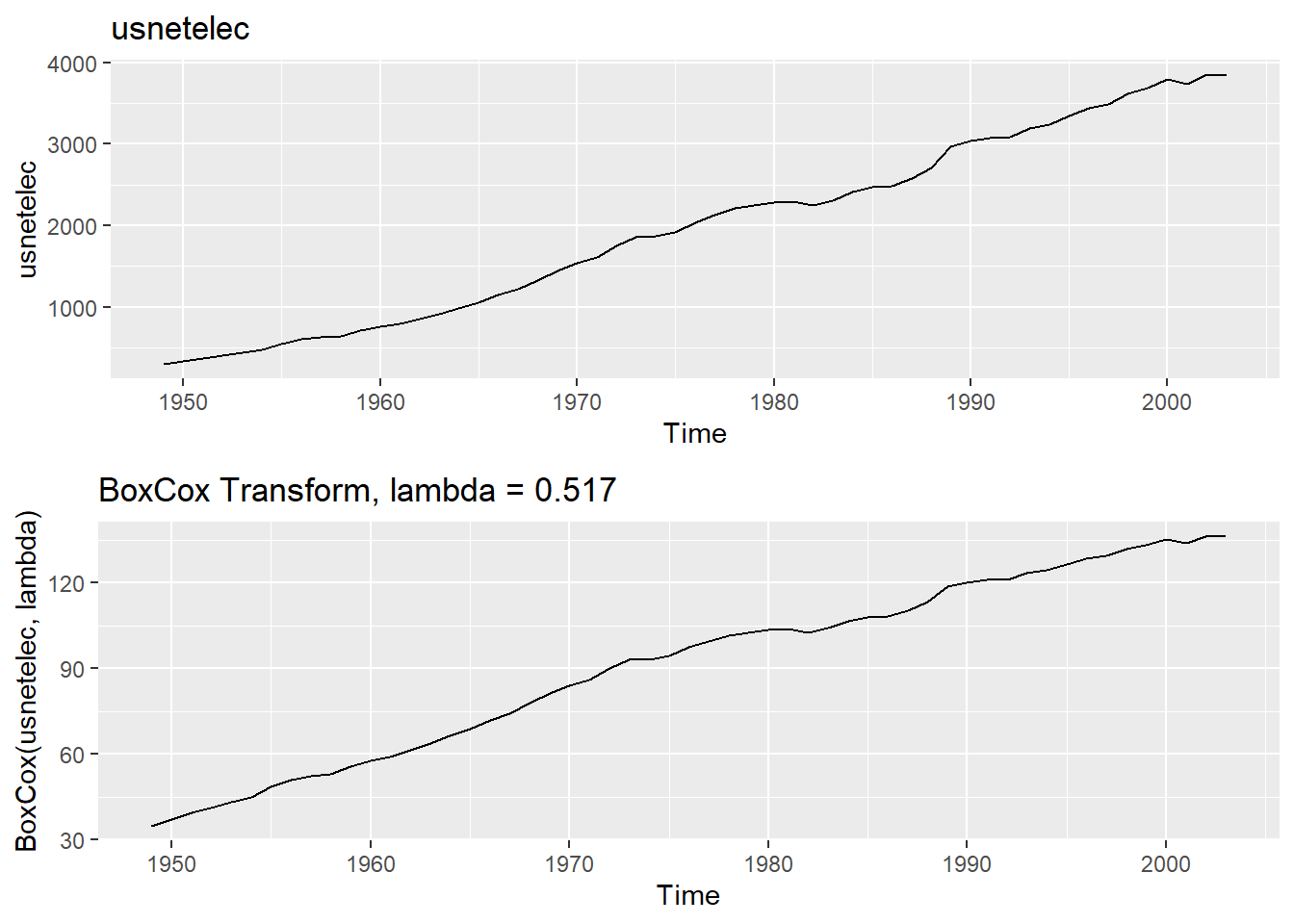#### usgdp

data(usgdp)
invisible(lambda <- round(BoxCox.lambda(usgdp),3))
grid.arrange(autoplot(usgdp) + ggtitle("usgdp"),autoplot(BoxCox(usgdp,lambda)) + ggtitle(paste("BoxCox Transform, lambda =", lambda)), ncol = 1)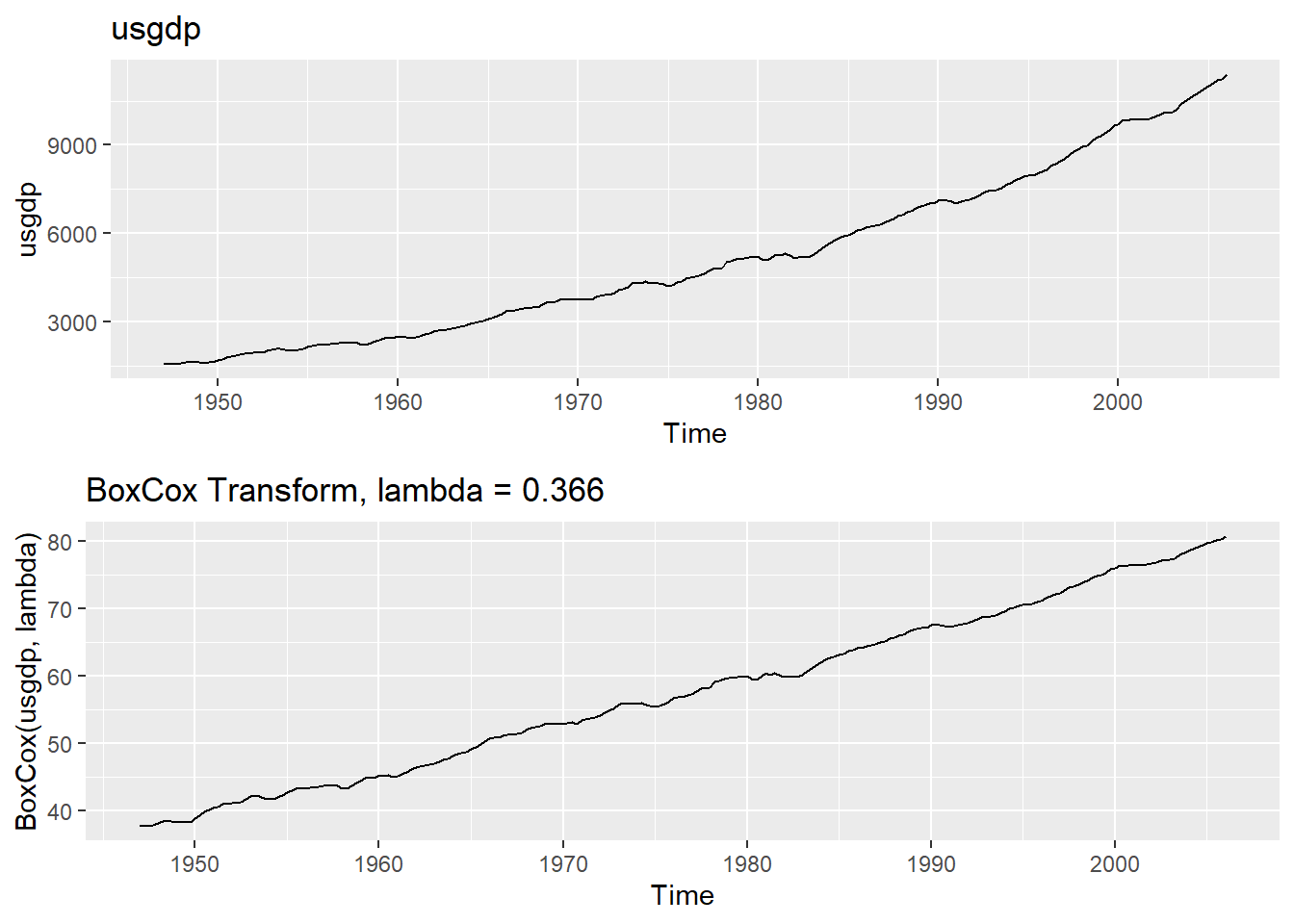#### mcopper

data(mcopper)
invisible(lambda <- round(BoxCox.lambda(mcopper),3))
grid.arrange(autoplot(mcopper) + ggtitle("mcopper"),autoplot(BoxCox(mcopper,lambda)) + ggtitle(paste("BoxCox Transform, lambda =", lambda)), ncol = 1)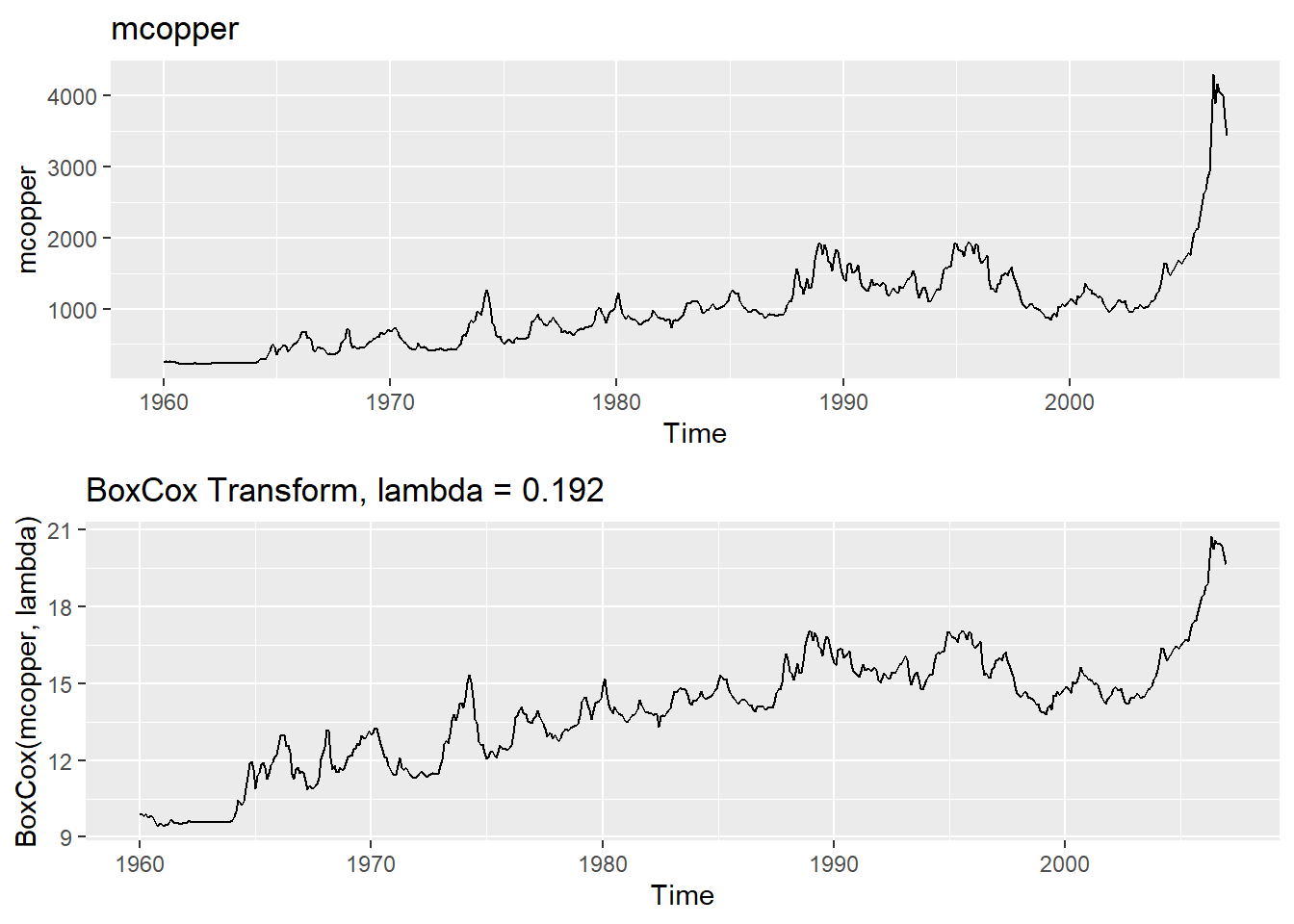#### enplanements

data(enplanements)
invisible(lambda <- round(BoxCox.lambda(enplanements),3))
grid.arrange(autoplot(enplanements) + ggtitle("enplanements"),autoplot(BoxCox(enplanements,lambda)) + ggtitle(paste("BoxCox Transform, lambda =", lambda)), ncol = 1)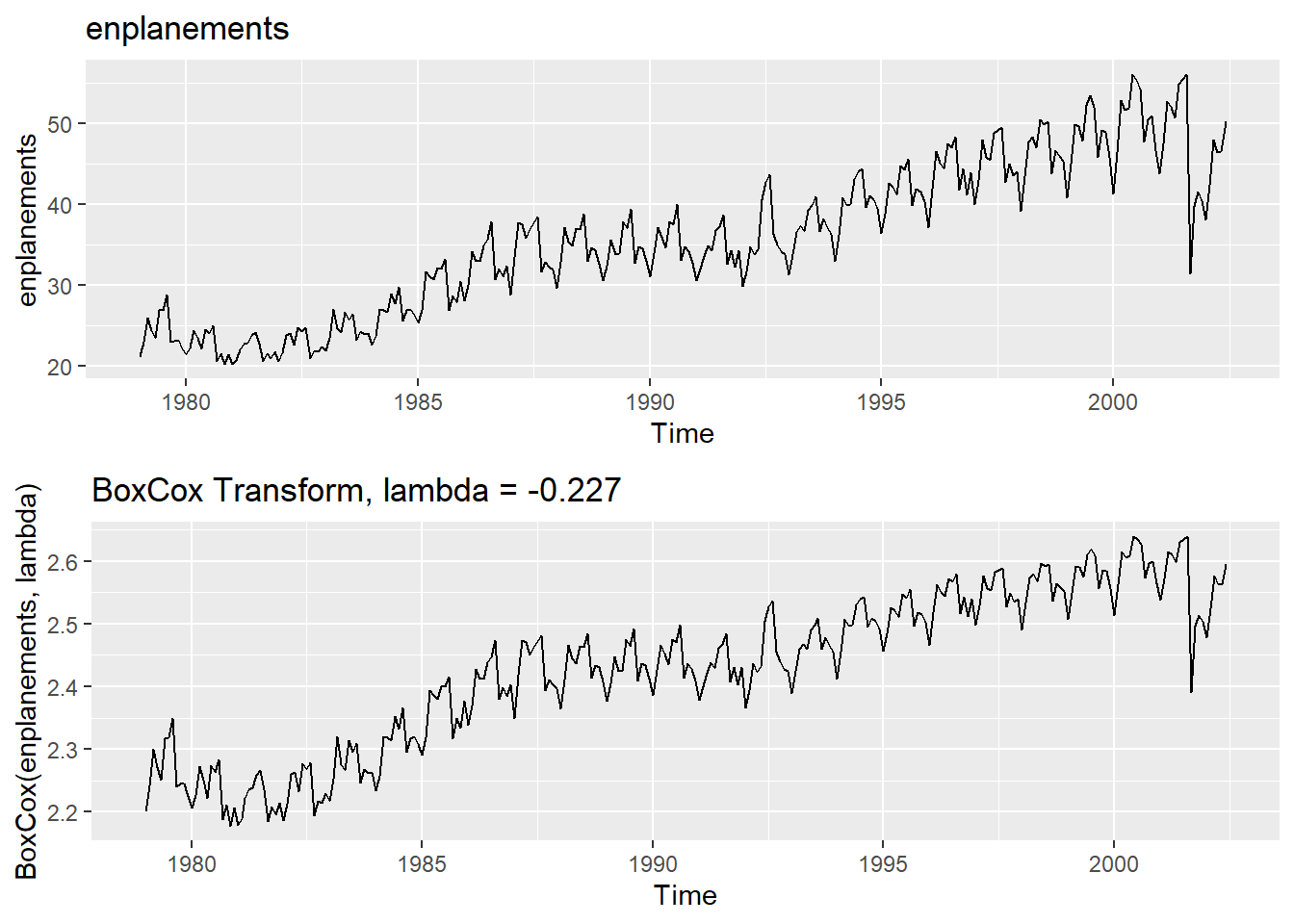## Question 3.2

Why is a Box-Cox transformation unhelpful for the cangas data?

cangas is Monthly Canadian gas production, billions of cubic metres, January 1960 - February 2005

data(cangas)
invisible(lambda <- round(BoxCox.lambda(cangas),3))
grid.arrange(autoplot(cangas) + ggtitle("cangas"),autoplot(BoxCox(cangas,lambda)) + ggtitle(paste("BoxCox Transform, lambda =", lambda)), ncol = 1)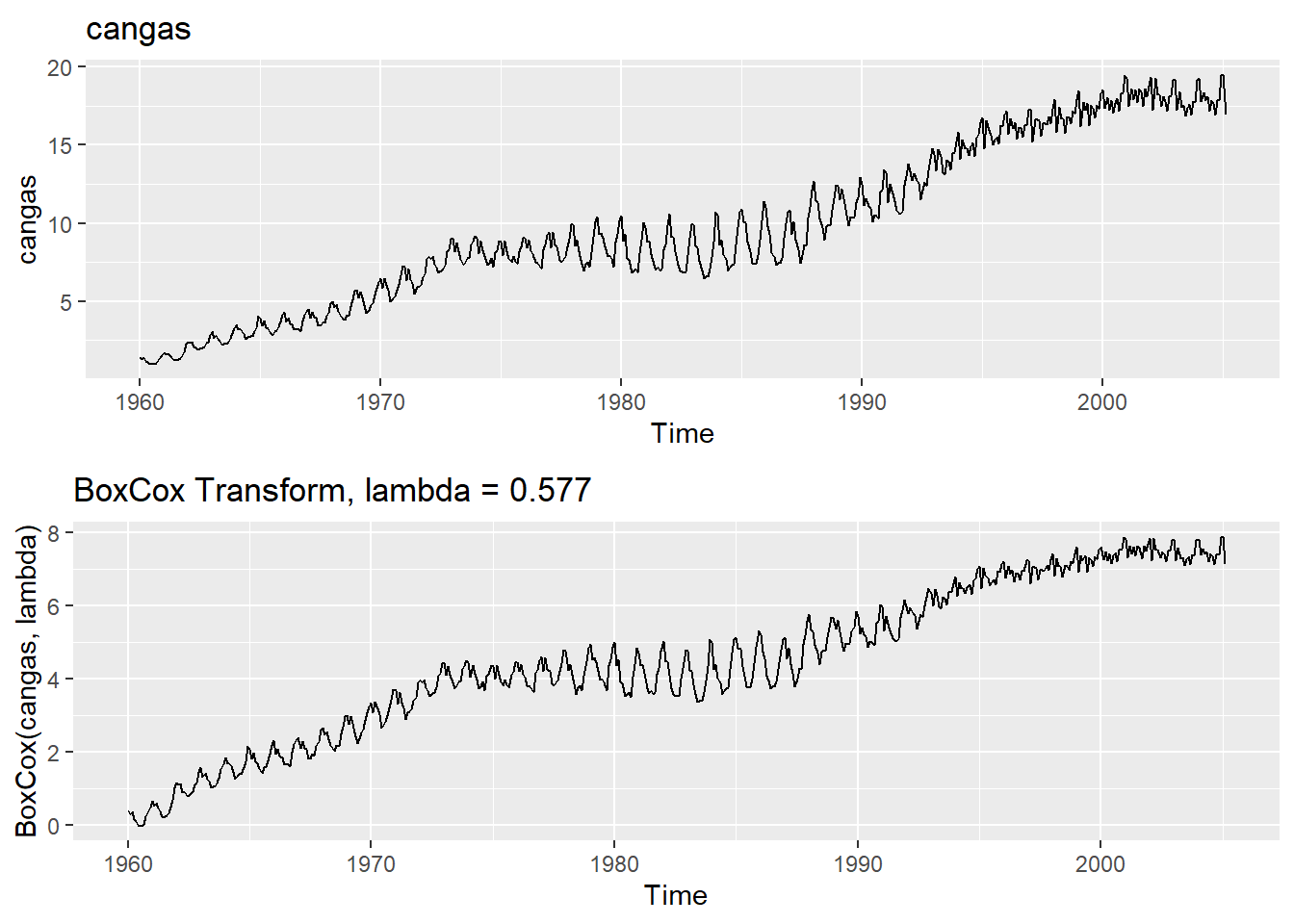The variability issue in this time series is still the same after the BoxCox transformation (low variability after 1995, high variability between 1980-1990). The other time series did not have evidence of cyclic patterns, but this time series does. The cyclic pattern in this time series causes changes in its variability. In addition, there are situations where variation is not extreme enough for a transformation to be needed.

## Question 3.3

What Box-Cox transformation would you select for your retail data (from Exercise 3 in Section 2.10)?

I would use a Box-Cox transformation with lambda value of 0.214. This value successfully stabilizes the time series.

temp = tempfile(fileext = ".xlsx")
dataURL <- "https://otexts.com/fpp2/extrafiles/retail.xlsx"
myts <- ts(retaildata[,"A3349396W"], frequency=12, start=c(1982,4))
invisible(lambda <- round(BoxCox.lambda(myts),3))
grid.arrange(autoplot(myts) + ggtitle("myts"),autoplot(BoxCox(myts,lambda)) + ggtitle(paste("BoxCox Transform, lambda =", lambda)), ncol = 1)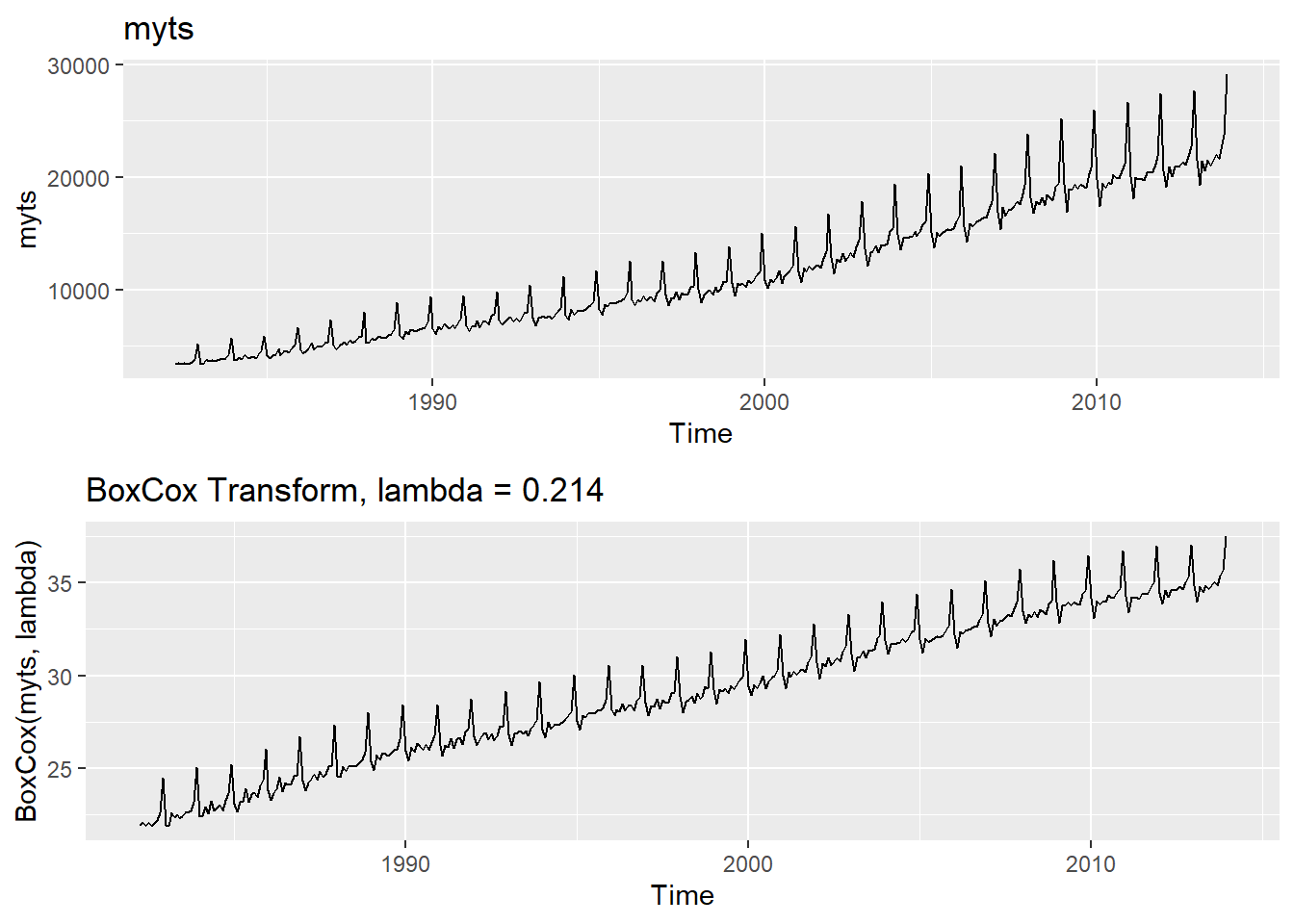## Question 3.8

For your retail time series (from Exercise 3 in Section 2.10):

1. Split the data into two parts using
myts.train <- window(myts, end=c(2010,12))
myts.test <- window(myts, start=2011)
1. Check that your data have been split appropriately by producing the following plot.
autoplot(myts) +
autolayer(myts.train, series="Training") +
autolayer(myts.test, series="Test")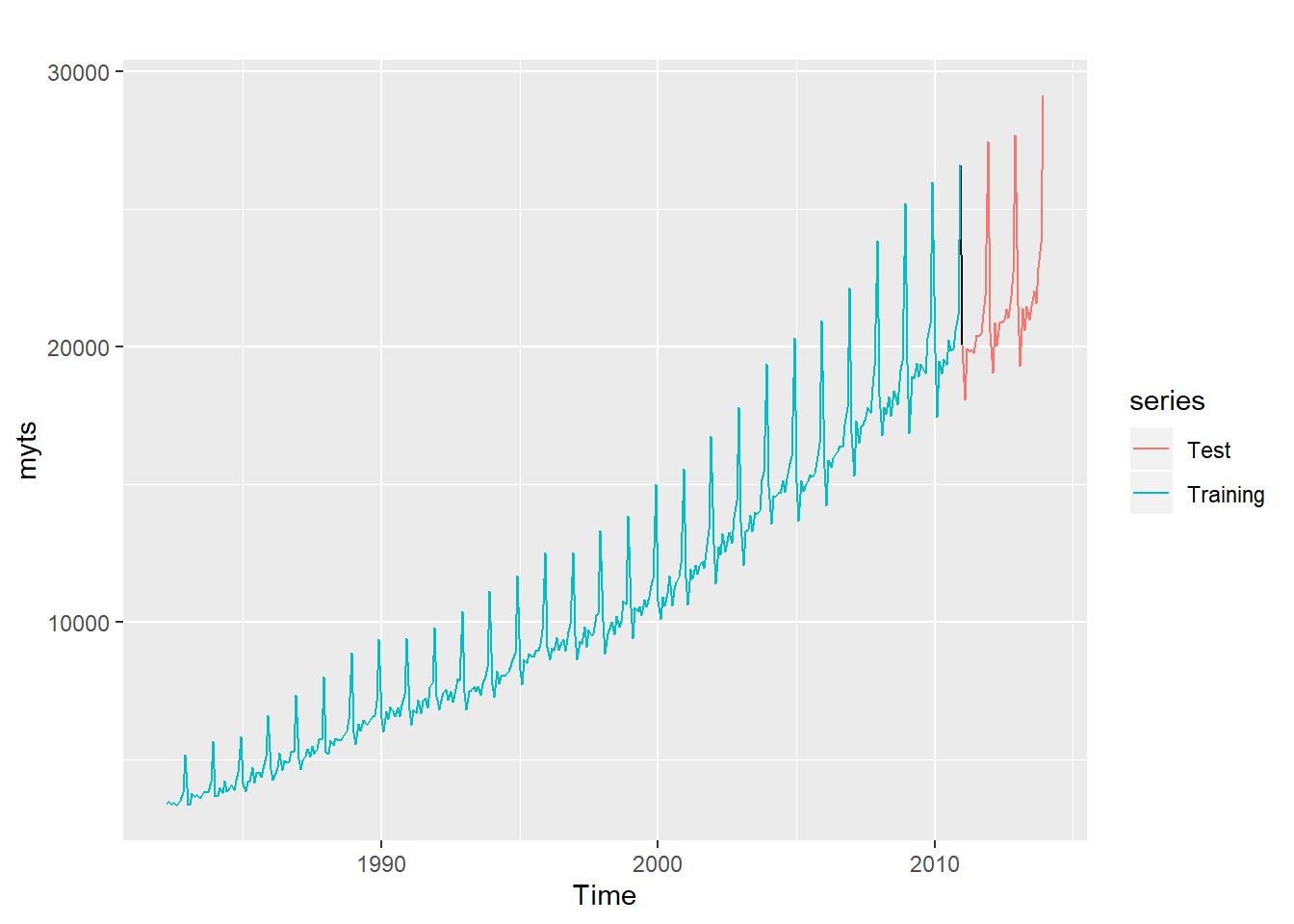1. Calculate forecasts using snaive applied to myts.train.
fc <- snaive(myts.train)
1. Compare the accuracy of your forecasts against the actual values stored in myts.test.

All error measures for the test set are higher than error measures for the training set. Since the values in the test dataset are > 20,000, an RMSE of 983 is a decent result.

accuracy(fc,myts.test)
##                    ME     RMSE      MAE      MPE     MAPE     MASE
## Training set 598.4838 699.2360 601.5060 5.961516 5.997664 1.000000
## Test set     882.2833 982.8526 882.2833 4.172002 4.172002 1.466791
##                   ACF1 Theil's U
## Training set 0.5972618        NA
## Test set     0.5627543 0.4906103
1. Check the residuals.
checkresiduals(fc)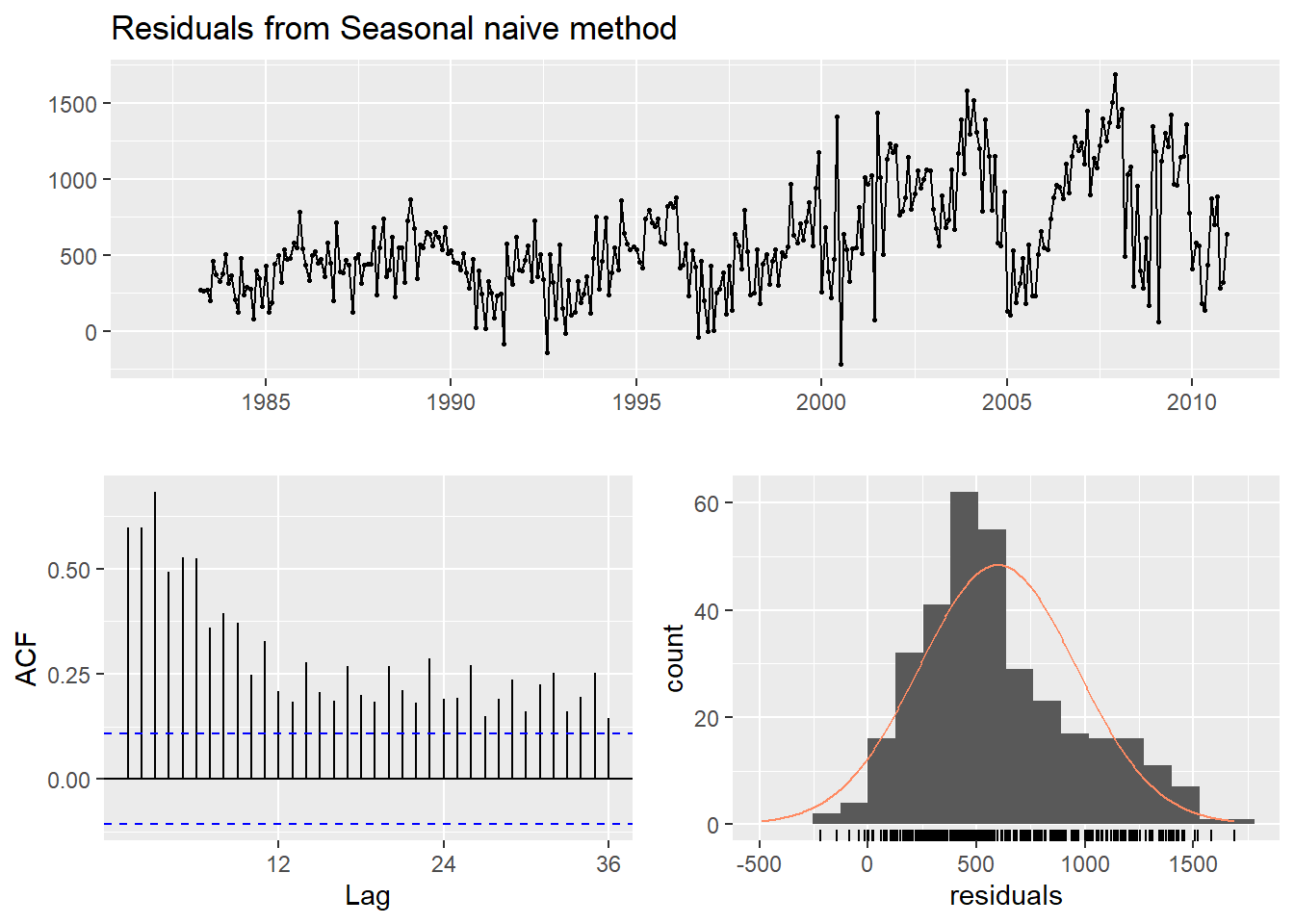##
##  Ljung-Box test
##
## data:  Residuals from Seasonal naive method
## Q* = 1101, df = 24, p-value < 2.2e-16
##
## Model df: 0.   Total lags used: 24

Do the residuals appear to be uncorrelated and normally distributed?

The residuals are not normally distributed (there is right skew), with more residual variability in the test set than the training set. The lag shows that residuals are not uncorrelated.

1. How sensitive are the accuracy measures to the training/test split?

We can test training/test set split sensitivity using cross validation.

modelcv <- CVar(myts, k=5, lambda=0.15)
autoplot(myts, series="Data") +
autolayer(modelcv$testfit, series="Fits") + autolayer(modelcv$residuals, series="Residuals")
## Warning: Removed 12 rows containing missing values (geom_path).

## Warning: Removed 12 rows containing missing values (geom_path).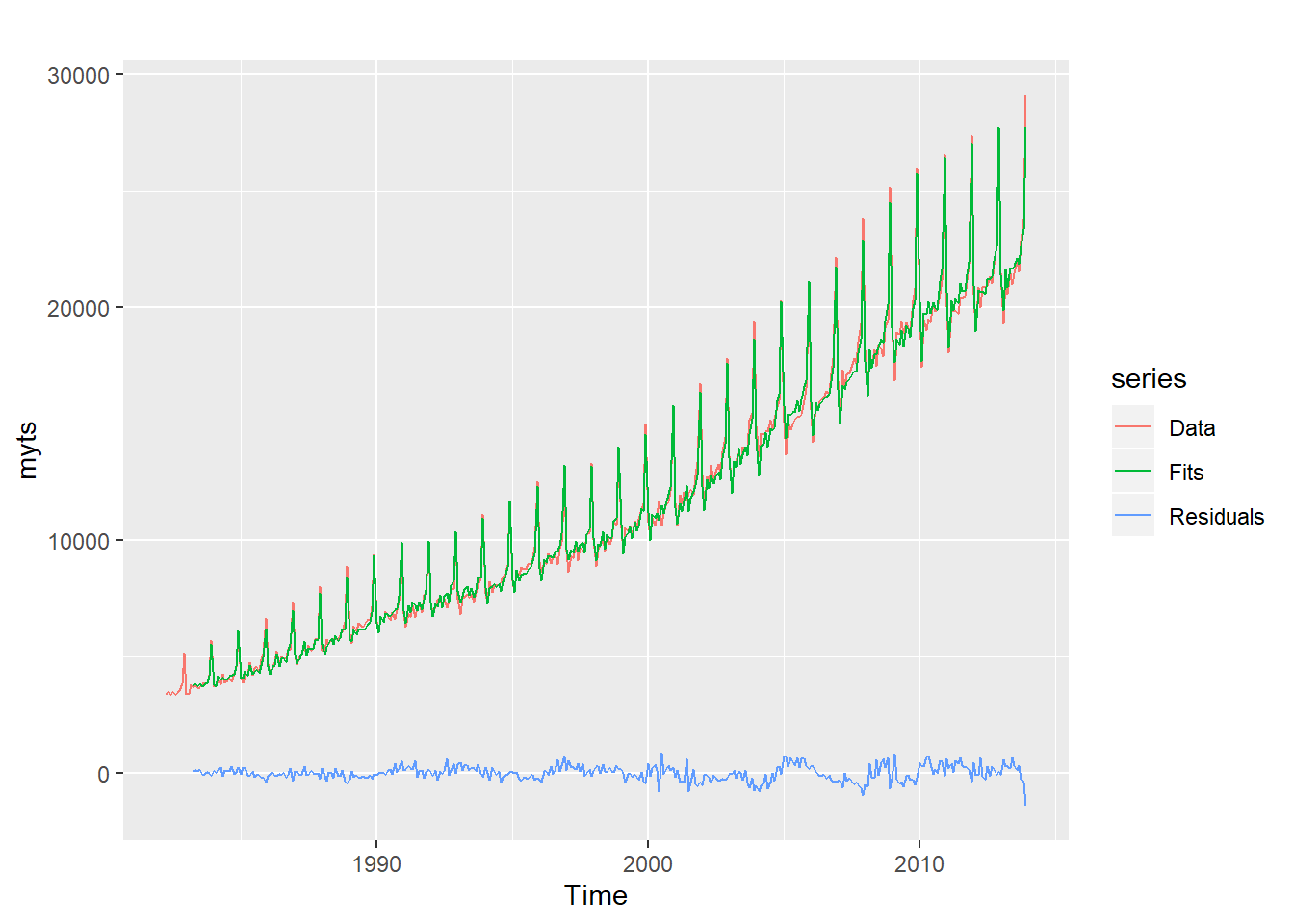ggAcf(modelcv\$residuals)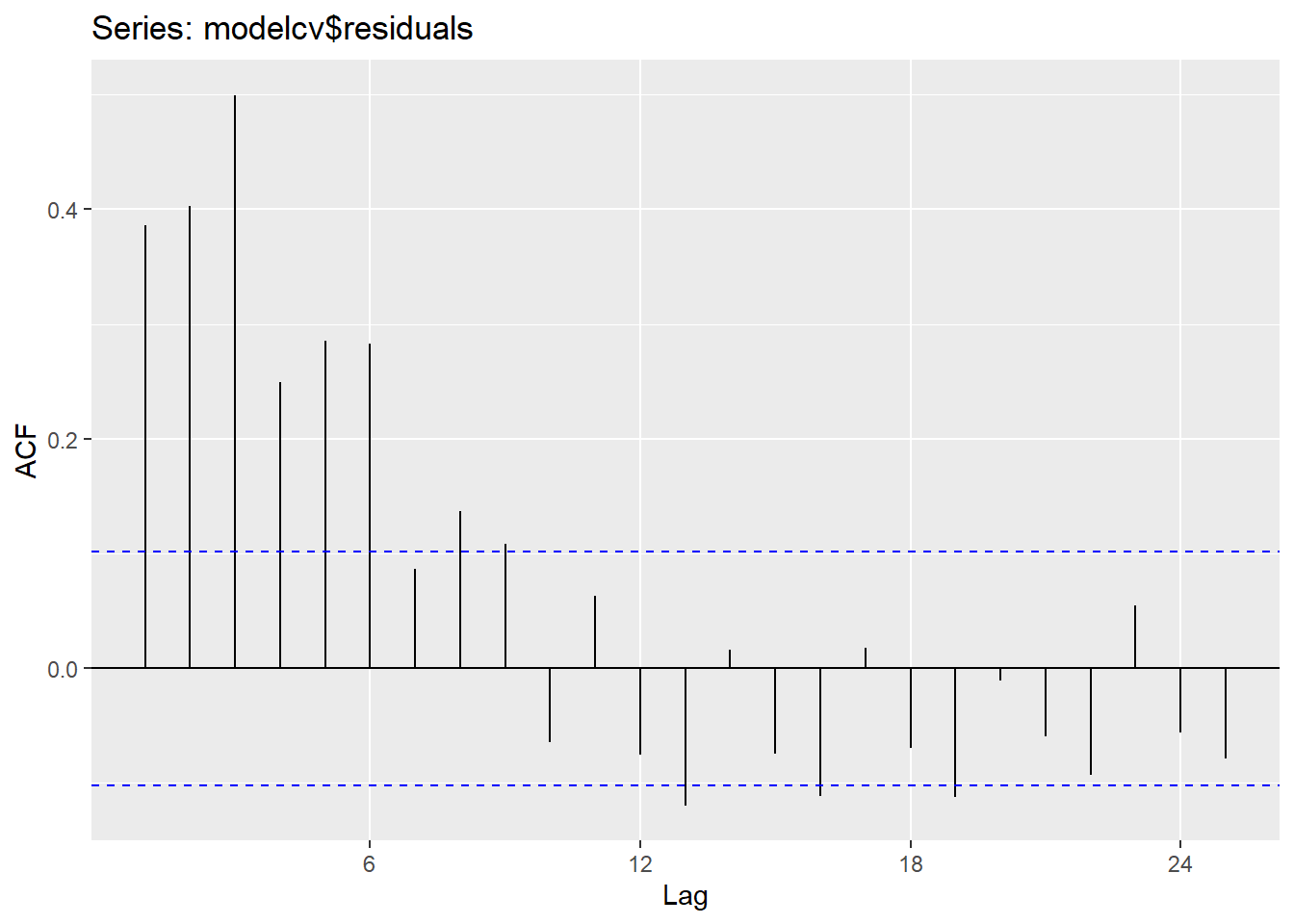What would happen if we changed the train and test size? Let’s create a new test train split and test the error.

The error did not change much when moving back the split by 6 months, but moving the split forward increased the error for the test set.

myts.train <- window(myts, end=c(2010,6))
myts.test <- window(myts, start=c(2010,7))
fc <- snaive(myts.train)
accuracy(fc,myts.test)
##                    ME     RMSE      MAE      MPE     MAPE     MASE
## Training set 598.1312 699.8920 601.2089 6.017075 6.053886 1.000000
## Test set     890.4333 984.3877 890.4333 4.278399 4.278399 1.481072
##                   ACF1 Theil's U
## Training set 0.6020708        NA
## Test set     0.4854022 0.4236731
myts.train <- window(myts, end=c(2011,6))
myts.test <- window(myts, start=c(2011,7))
fc <- snaive(myts.train)
accuracy(fc,myts.test)
##                    ME      RMSE      MAE      MPE     MAPE     MASE
## Training set 596.4979  696.4529 599.4667 5.900267 5.935776 1.000000
## Test set     962.1125 1042.9384 962.1125 4.487378 4.487378 1.604947
##                   ACF1 Theil's U
## Training set 0.5919533        NA
## Test set     0.3404947 0.4572131